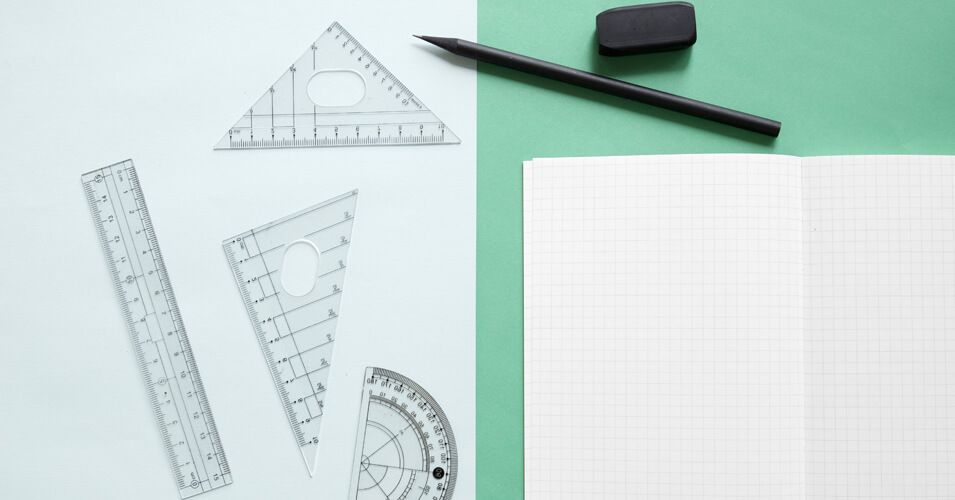• Save

# Coordinate Geometry Questions and Answers

Last month 322 Views
NEWQ :

If (2, 0) is a solution of the linear equation 2x + 3y = 5, then the value of k is:

(A) 2

(B) 4

(C) 6

(D) 5

Q :

If a linear equation is of the form x = k, where k is a constant, then graph of the equation will be

(A) a line making positive acute angle with x - axis

(B) a line parallel to y - axis

(C) a line parallel to x - axis

(D) a line cutting both the axes

Q :

The point Q(a, b ) is first reflected in y-axis to Q1 and Q1 is reflected in x-axis to (-5,3 ). The co-ordinates of point Q are

(A) (-5 , -3)

(B) ( - 5, 3 )

(C) ( 5 , -3 )

(D) ( 5, 3 )

Q :

Point A (2, 1) divides segment BC in the ratio 2 : 3. Co-ordinates of B are (1, -3 ) and C are (4 , y). What is the value of y ?

(A) -8

(B) 7

(C) 8

(D) -7

Q :

The graph of the equations 25x + 75y = 225 and x = 9 meet the point:

(A) ( 3, 0 )

(B) (0, 3)

(C) (0, 9)

(D) (9, 0)

Q :

Point P is the midpoint of segment AB. Co-ordinates of point Pare (2,1) and point B are (11,5) Co-ordinates of point A are

(A) ( 6.5, 3 )

(B) ( 7, 3 )

(C) ( -7, -3)

(D) ( -6.5 , -3 )

Q :

The length of the portion of the straight line 3x + 4y = 12 intercepted between the axes is :

(A) 4

(B) 7

(C) 5

(D) 3

Q :

The area bounded by the lines x = 0, y = 0, x + y = 1, 2x + 3y = 6 (in square units) is

(A) $$2{1\over2}$$

(B) 3

(C) 2

(D) $$2{1\over3}$$

Q :

What is the equation of the line whose y-intercept is -3/4 and making an angle of 45 ° with the positive x-axis?

(A) 4x - 4y = -3

(B) 3x - 3y = 4

(C) 4x - 4y = 3

(D) 3x - 3y = -4

Q :

The graph of 3x + 4y - 24 = 0 forms a triangle OAB with the coordinate axes, where O is the origin. Also, the graph of x + y + 4 = 0 forms a triangle OCD with the coordinate axes. Then the area of ∆ OCD is equal to:

(A) $${2\over3}$$ of area of ∆ OAB

(B) $${2\over5}$$ of area of ∆ OAB

(C) $${1\over2}$$ of area of ∆ OAB

(D) $${1\over3}$$ of area of ∆ OAB